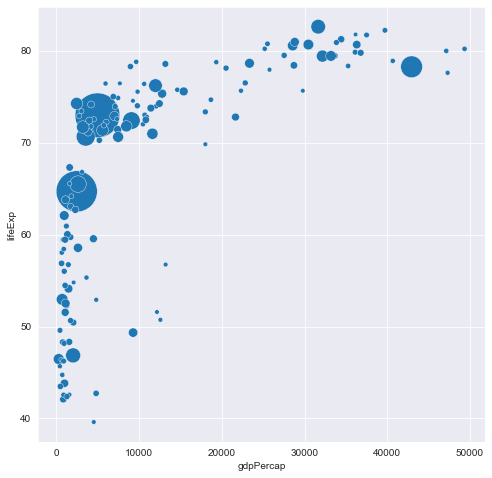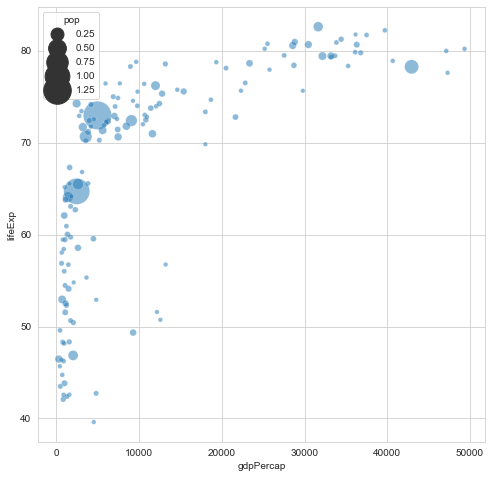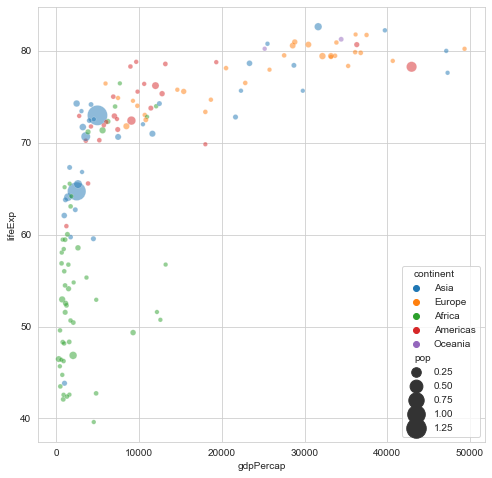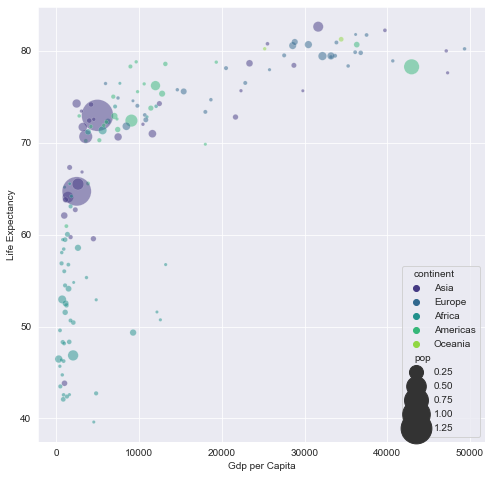## Most Basic Bubble Chart

A bubble plot is basically a scatterplot with an additional dimension: size of points. Using seaborn library, a bubble plot can be constructed using the `scatterplot()` function. In the example, the following parameters are used to build a basic bubble plot:

• `data` : Input data structure
• `x` : The data position on the x axis
• `y` : The data position on the y axis
• `size` : Grouping variable that will produce points with different sizes
• `alpha` : Transparancy ratio

Here, the gapminder data set is used and the relationship between life expectancy (y) and gdp per capita (x) of world countries is represented. The population of each country is represented through dot size.

Note: the `gapminder` dataset can be installed with `pip install gapminder`

``````# libraries
import matplotlib.pyplot as plt
import seaborn as sns
from gapminder import gapminder # import data set

# Control figure size for this notebook:
plt.rcParams['figure.figsize'] = [8, 8]

# data
data = gapminder.loc[gapminder.year == 2007]

# use the scatterplot function to build the bubble map
sns.scatterplot(data=data, x="gdpPercap", y="lifeExp", size="pop", legend=False, sizes=(20, 2000))

# show the graph
plt.show()``````## Control Bubble Size

You can control the sizes of bubbles using the `sizes` argument. You should pass the minimum and maximum size to use such that other values are normalized within this range.

``````# libraries
import matplotlib.pyplot as plt
import seaborn as sns
from gapminder import gapminder # import data set

# data
data = gapminder.loc[gapminder.year == 2007]

# use the scatterplot function
sns.scatterplot(data=data, x="gdpPercap", y="lifeExp", size="pop", alpha=0.5, sizes=(20, 800))

# show the graph
plt.show()``````## Bubble color

We added a third dimension to our scatterplot with the sizes of bubbles. Now, we will add a forth dimension by mapping colors to variables. Here, the continent of each country is used to control bubble color and passed to the function with the `hue` argument.

``````# use the scatterplot function
sns.scatterplot(data=data, x="gdpPercap", y="lifeExp", size="pop", hue="continent", alpha=0.5, sizes=(20, 400))

# show the graph
plt.show()``````## Control Aestethics

You can set the theme using the `set_style()` function of matplotlib and change the color palette of bubbles passing the `palette` argument to the `scatterplot()` function. It is also recomended to take the legend outside of the plot using the `legend()` function.

``````# set seaborn "whitegrid" theme
sns.set_style("darkgrid")

# use the scatterplot function
sns.scatterplot(data=data, x="gdpPercap", y="lifeExp", size="pop", hue="continent", palette="viridis", edgecolors="black", alpha=0.5, sizes=(10, 1000))

# Add titles (main and on axis)
plt.xlabel("Gdp per Capita")
plt.ylabel("Life Expectancy")

# Locate the legend outside of the plot
# plt.legend(bbox_to_anchor=(1, 1), loc='upper left', fontsize=17)

# show the graph
plt.show()``````## Contact & Edit

👋 This document is a work by Yan Holtz. You can contribute on github, send me a feedback on twitter or subscribe to the newsletter to know when new examples are published! 🔥